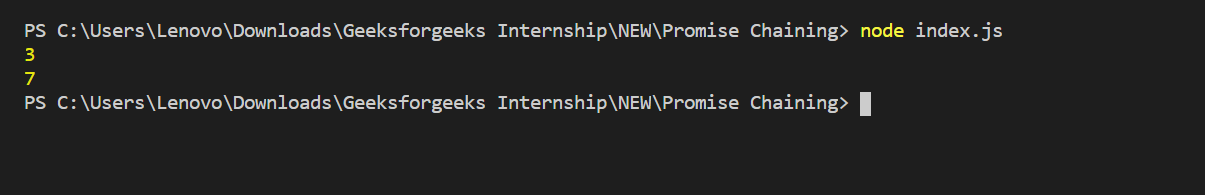Open in App
Not now

# Node.js Promise Chaining

• Last Updated : 08 Oct, 2021

Promise chaining: Promise chaining is a syntax that allows you to chain together multiple asynchronous tasks in a specific order. This is great for complex code where one asynchronous task needs to be performed after the completion of a different asynchronous task.

To demonstrate promise chaining, the following function will be used to simulate an asynchronous task. In reality, it’s just adding up a couple of numbers, waiting two seconds, and fulfilling the promise with the sum.

Filename: index.js

 `const add = (a, b) => {      ` `    ``return` `new` `Promise((resolve, reject) => {         ` `        ``setTimeout(() => {             ` `            ``if` `(a < 0 || b < 0) {                  ` `                ``return` `reject(``'Numbers must be non-negative'``) ` `            ``}  ` `            ``resolve(a + b)          ` `        ``}, 2000)  ` `    ``}) ` `} ` ` `  `add(1, 2).then((sum) => {      ` `    ``console.log(sum)  ``// Print 3    ` `    ``return` `add(sum, 4) ` `}).then((sum2) => {      ` `    ``console.log(sum2) ``// Print 7  ` `}).``catch``((e) => {  ` `    ``console.log(e)  ` `}); `

Step to run the program:

• Run index.js file using below command:
`node index.js`With the dummy asynchronous function defined, promise chaining can be used to call add twice. The code below adds up 1 and 2 for a total of 3. It then uses the sum value 3 as the input for another call to add. The second call to add adds up 3 and 4 for a total of 7.

Promise chaining occurs when the callback function returns a promise. It allows you to chain on another then call which will run when the second promise is fulfilled. Catch can still be called to handle any errors that might occur along the way.

My Personal Notes arrow_drop_up
Related Articles